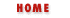#Chapter 15Perl Quick Reference## 15.8 Arithmetic Functions

abs expr(dagger)

Returns the absolute value of its operand.

atan2 y, x

Returns the arctangent of y/x in the range -pi to pi.

cos expr(dagger)

Returns the cosine of expr (expressed in radians).

exp expr(dagger)

Returns e to the power of expr.

int expr(dagger)

Returns the integer portion of expr.

log expr(dagger)

Returns natural logarithm (base e) of expr.

rand [ expr ]

Returns a random fractional number between 0 and the value of expr. If expr is omitted, returns a value between 0 and 1.

sin expr(dagger)

Returns the sine of expr (expressed in radians).

sqrt expr(dagger)

Returns the square root of expr.

srand [ expr ]

Sets the random number seed for the rand operator.

time

Returns the number of seconds since January 1, 1970. Suitable for feeding to gmtime and localtime.Object-Oriented ProgrammingConversion Functions